##### Print Instructions

NOTE: Only your test content will print.
To preview this test, click on the File menu and select Print Preview.

See our guide on How To Change Browser Print Settings to customize headers and footers before printing.

# Data Analysis - Statistical Representation (Continuing Education)

Print Test (Only the test content will print)

## Data Analysis - Statistical Representation

1.
Select the best description for the statistical representation in the image below.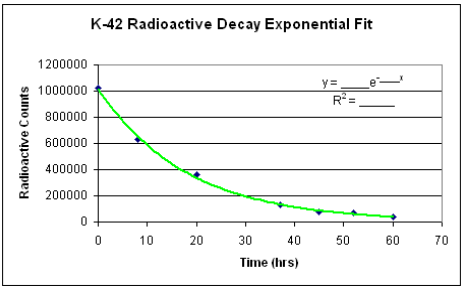1. Mean Shift
2. Redistribution
3. Stability
4. Trend
5. Variation
2.
Select the best description for the statistical representation in the image below.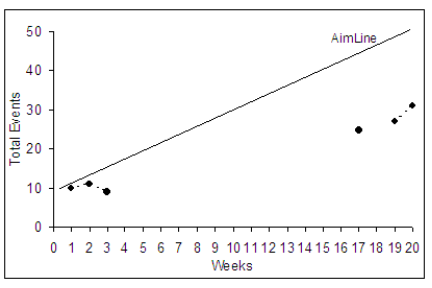1. Missing Data
2. Outlier
3. Redistribution
4. Stability
5. Trend
3.
Select the best description for the statistical representation in the image below.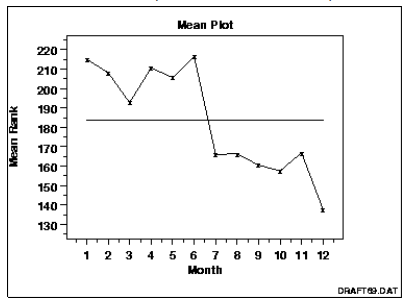1. Mean Shift
2. Outlier
3. Redistribution
4. Stability
5. Trend
4.
Select the best description for the statistical representation in the image below.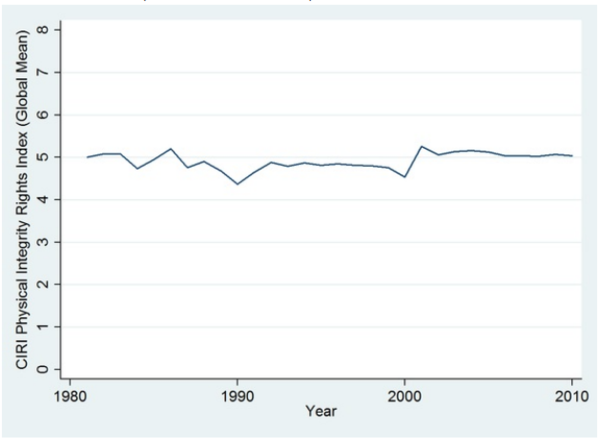1. Missing Data
2. Outlier
3. Redistribution
4. Stability
5. Variation
5.
Select the best description for the statistical representation in the image below.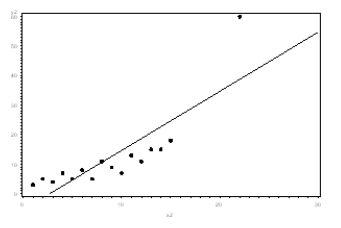1. Mean Shift
2. Missing Data
3. Outlier
4. Stability
5. Variation
6.
Select the best description for the statistical representation in the image below.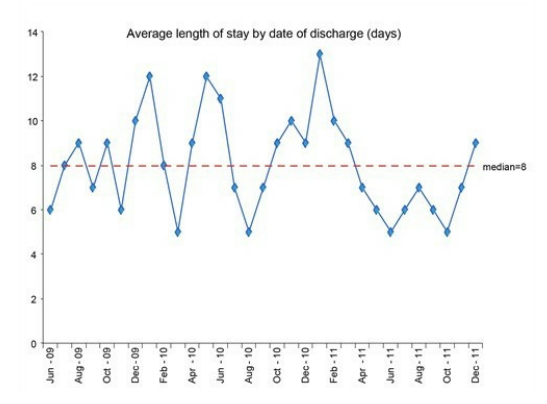1. Mean Shift
2. Redistribution
3. Stability
4. Trend
5. Variation
7.
A hospital wants to keep track of the number of people who are treated for the flu in its emergency department. This is an example of which of the following data types?
1. Categorical
2. Continuous
3. Discrete
4. Ordinal
8.
A company wants to keep track of the amount of gas used in its delivery trucks. This is an example of which of the following data types?
1. Categorical
2. Continuous
3. Discrete
4. Ordinal
9.
A company wants to keep track of the age and sex of its customers. This is an example of which of the following data types?
1. Categorical
2. Continuous
3. Discrete
4. Ordinal
10.
A company asks its customers to rank its customer service on a 10 point scale. This is an example of which of the following data types?
1. Categorical
2. Continuous
3. Discrete
4. Ordinal
11.
Which of the following types of graphs would be the best choice for displaying the data in the image below? Hint: there is only one variable.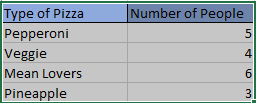1. Bar Graph
2. Circle Graph
3. Histogram
4. Line Graph
5. Scatter Plot
12.
Which of the following types of graphs would be the best choice for displaying the data in the image below?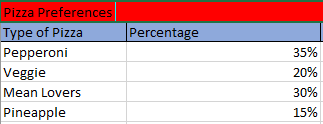1. Bar Graph
2. Circle Graph
3. Histogram
4. Line Graph
5. Scatter Plot
13.
Which of the following types of graphs would be the best choice for displaying the data in the image below?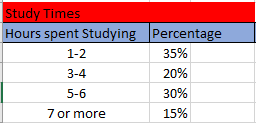1. Bar Graph
2. Circle Graph
3. Histogram
4. Line Graph
5. Scatter Plot
14.
Which of the following types of graphs would be the best choice for displaying the data in the image below?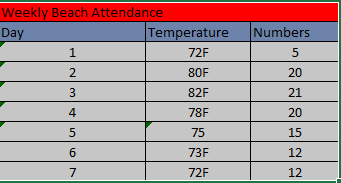1. Bar Graph
2. Circle Graph
3. Histogram
4. Line Graph
5. Scatter Plot
15.
Which of the following types of graphs would be the best choice for displaying the data in the image below?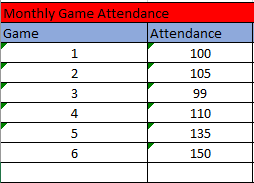1. Bar Graph
2. Circle Graph
3. Histogram
4. Line Graph
5. Scatter PlotYou need to be a HelpTeaching.com member to access free printables.